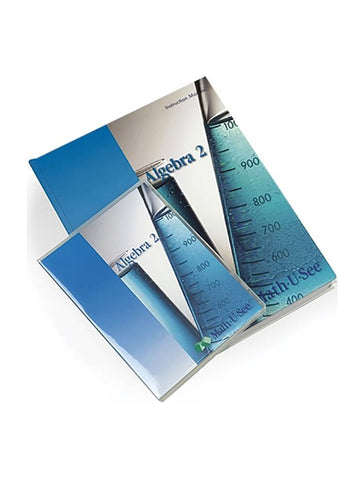# Math-U-See Algebra 2 Instruction Pack

• \$ 97.00

#### Algebra 2 Instruction Pack contains the instruction manual with lesson-by-lesson instructions and detailed solutions, and the DVD with lesson-by-lesson video instruction. Factoring polynomials, quadratic formula, graphing conic sections and other algebra 2 topics.

Major Concepts and Skills Include:

• Simplifying multiple-degree rational expressions
• Working with imaginary and complex numbers
• Understanding and applying the binomial theorem
• Solving basic quadratic equations with factoring
• Applying analytic geometry to conic sections
• Solving simultaneous equations (linear and conic) using graphing, substitution, and elimination